## Topic 6.3: Transformations to Linear Regression (HOWTO)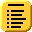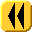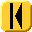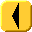Introduction Notes Theory HOWTO Examples Engineering Error Questions Matlab Maple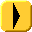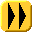# Problem

Given data (xi, yi), for i = 1, 2, ..., n which is known to approximate an exponential curve, find the best fitting exponential function of the form y(x) = aebx.

# Assumptions

We will assume the model is correct and that the data is defined by two vectors x = (xi) and y = (yi).

# Tools

We will use algebra and linear regression.

# Process

Take the logarithm of the y values and define the vector φ = (φi) = (log(yi)).

Now, find the least-squares curve of the form c1 x + c2 which best fits the data points (xiφi). See the Topic 6.1 Linear Regression.

Having found the coefficient vector c, the best fitting curve is

y = ec2 ec1 x.